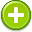#measurements 中文解釋 wordnet sense Collocation Usage
Noun
/ˈmeZHərmənt/,Font size:measurements, plural;
1. The action of measuring something
• - accurate measurement is essential
• - a telescope with which precise measurements can be made
2. The size, length, or amount of something, as established by measuring
• - his inseam measurement
3. A unit or system of measuring
• - a hand is a measurement used for measuring horses

1. (measured) mensural: having notes of fixed rhythmic value
2. (measured) the rhythmic arrangement of syllables
3. (measured) deliberate: carefully thought out in advance; "a calculated insult"; "with measured irony"
4. (measuring) measurement: the act or process of assigning numbers to phenomena according to a rule; "the measurements were carefully done"; "his mental measurings proved remarkably accurate"
5. (Measurement (intellect)) Measuring the Intellect is the mathematical model for measuring the intellectual power in the process of creating the Intellectual Product by Intelligent Systems. The Hypothesis and its practical applications is proposed by Professor Vladimir Brandin.
6. (Measurement (quantum mechanics)) The measurement problem in quantum mechanics is the unresolved problem of how (or if) wavefunction collapse occurs. ...
7. (Measuring) In science, measurement is the process of estimating or determining the magnitude of a quantity, such as length or mass, relative to a unit of measurement, such as a metre or a kilogram. ...
8. (measured) That has been determined by measurement; Deliberate but restrained; Rhythmically written in meter; metrical
9. (measurement) The act of measuring; Magnitude (or extent or amount) determined by measurement
10. (Measurement) The act or process of quantitatively comparing results with requirements.
11. (measurement) an estimated value that is, by its nature, subject to error. A person can count (an absolute value) one hundred beans and get the same quantity as someone else counting one hundred beans. ...
12. (Measurement) The act of obtaining knowledge about an event or characteristic through measured quantification or data.
13. (Measurement) A procedure for assigning a number to an object or an event.
14. (Measurement) Same as "process variable."
15. Measurement is the collection of quantitative data. Measurement involves comparison of the quantity of interest with a standard called a unit. The comparison is never perfect. As a result, measurements always include error. ...
16. (measurement) The process of assigning a number or category to an entity to describe an attribute of that entity. [ISO 14598]
17. (Measurement) The assignment of labels or numerals to the properties or attributes of observations, events, or objects according to specific rules.
18. (Measurement) means a repeatable, objective procedure for generating a measure.
19. ("A" Measurement) Or the size of the frame, it is measured in millimeters horizontally across the eyewire.  It is usually found on the temple or on the bridge.  Example: 52mm-18-140, usaully range from 40-60mm
20. "Measurement is the numerical estimation of the ratio of a magnitude of an attribute to a unit of the same attribute" (Michell, 1997, p383). Alternatively, it is a quality or quantity arrived at by observation of an operationalised measure of a variable in known circumstances.
21. (MEASUREMENT) Any method by which a rule or calibrated instrument on a scale of 1-100 is used to determine the quality, rate or vibration of an object. It can be also done by counting in whatever measurement is most suitable until a positive dowsing response is felt. ...
22. (Measurement) A survey');">quantity survey from plans or a field survey of work to be done.
23. (Measurement) A tool used to provide feedback to the learner and the trainer to determine where the learner is in relation to the ultimate goal or objective.
24. (Measurement) After a project is complete, the quantity of greenhouse gases still emitted is measured. This amount is subtracted from the baseline to confirm the predicted reduction.
25. (Measurement) An assertion that a transaction or event is recorded in the proper amount and in the proper period.